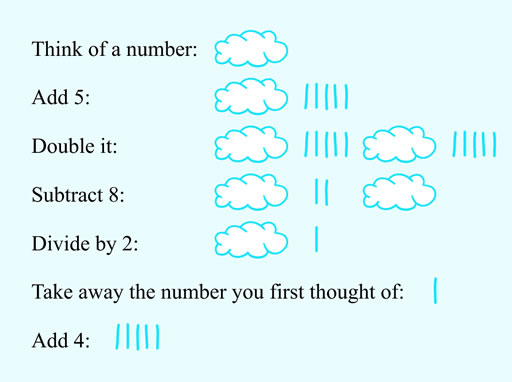Succeed with maths: part 2

Start this free course now. Just create an account and sign in. Enrol and complete the course for a free statement of participation or digital badge if available.

# 2.1 Understanding the number trick

One way to understand how the previous number trick works is to use a visual representation of each stage of the instructions, and that is how you are asked to approach this next activity. Take your time, and read over the instructions and hints carefully to start you off.

## Activity _unit5.2.2 Activity 5 How does this trick work?

Timing: Activity 5 How does this trick work?

To see how this trick works, read through the instructions from before. Because you could have thought of any number, replace this unknown number with a thought bubble like this:Keep the thought bubble as part of the new number that you get at each stage. So after the first step you will have something like this:+ IIIII (to represent 5).

### Discussion

When doubling, draw the cloud and associated number twice.

This is what our visualised representation of the number trick looks like:Figure _unit5.2.1 Figure 5 The number trick explained

This shows that the numerical answer is always going to be 5. It does not depend on which number was chosen first since there are no clouds, representing the initial number, involved in the final answer. So the letter of the alphabet chosen is always E. There are not many animals with names beginning with E and most people do think of an elephant first, but we’ve already thought about the pitfalls of that!

Rather than using a cloud to represent the number and explaining the trick visually, you could write this in a more mathematical way by using either a word or letter to represent the initial number. In maths unknown numbers are usually represented using a letter. Using ‘n’ to stand for this number the trick can be written out again without the clouds like this:

Table _unit5.2.2 Table 1 Showing an algebraic representation of the number trick
Instruction Expression
Think of a number
Double it

This can be re-written as

is the same as 2 lots of , so can be written in shorthand as

So

Subtract 8
Divide by 2

Dividing each part by 2 separately is the same as dividing the whole of , by 2. Taking this former approach helps us show the next step more clearly.

2 lots of n divided by 2 gives 1 lot of n, and clearly 2 divided by 2 gives 1. So, finally giving:

Take away the number you first thought of

This gives the same result, where n is not involved in the final answer. This time a more conventional mathematical representation was used rather than a cloud!

At first this may have appeared to be quite a daunting example, using both numbers and letters but hopefully you found that by working your way carefully through each stage you were able to understand how the final answer was obtained. Working step by step carefully through problems will really pay dividends as problems and concepts start to look more complicated. Remember this when completing the next activity with another number trick.